# Mimic Robot

ECE5725 Spring 2019 Final Project
Mark Li (mkl53) and Serena Krech (sk2282)

## Project Objective: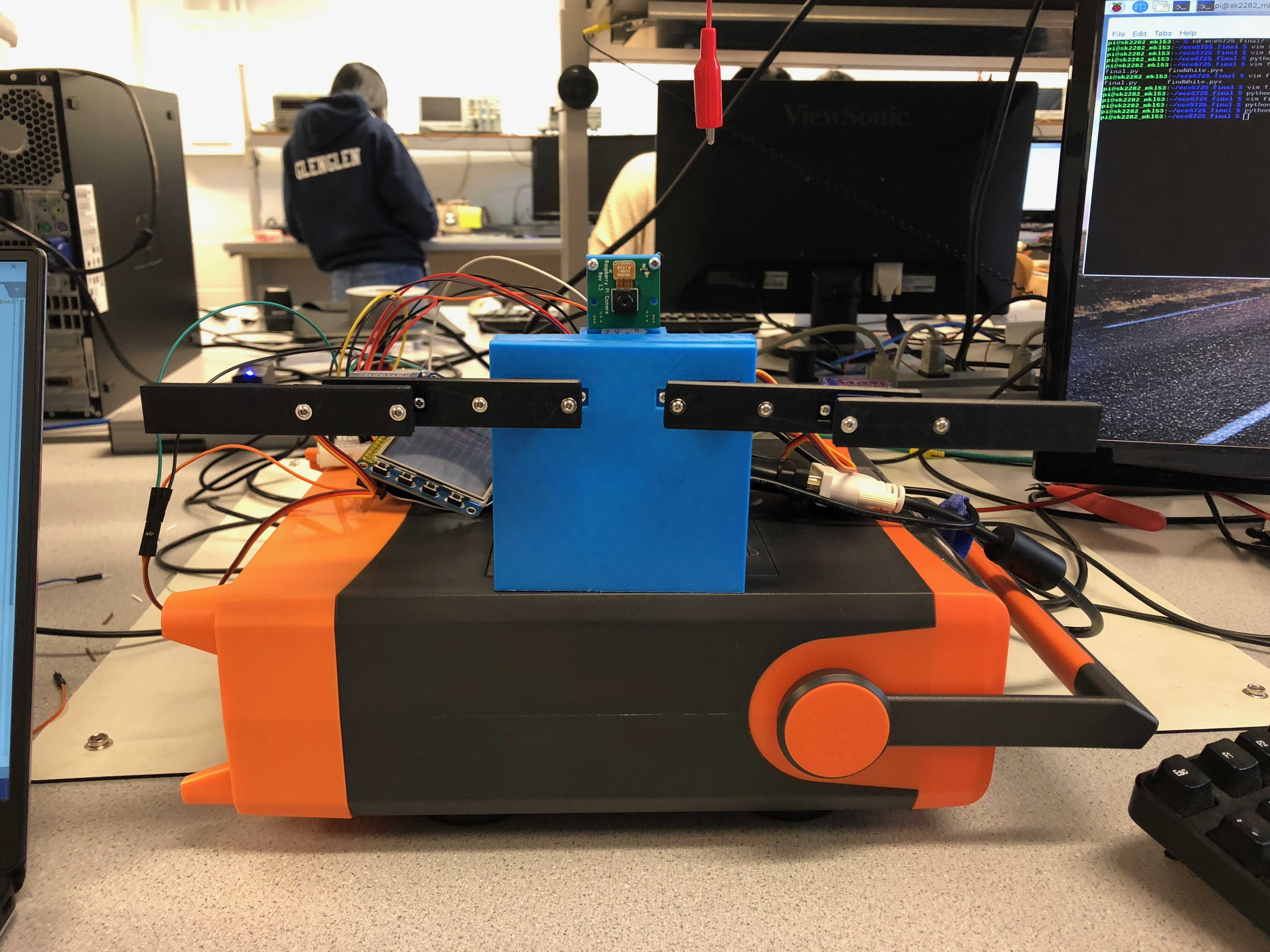• Detect location of hands and elbows
• Determine angles between these points
• Control servos to move arms
• Mimic human movement

## Introduction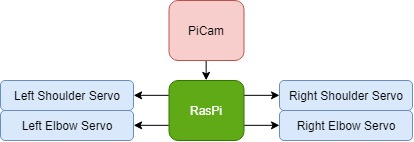In this project, we used a Raspberry Pi to process images and control servos to create a small robot that would mimic a person's movements. The RasPi recieved images as input from a PiCam and processed them in real time. We used OpenCV to identify the person as well as find their chest, elbow, and arm points. These points were then used to find angles between the upper arm and forearm as well as the angle of the upper arm at the shoulder. With this angle, we were able to map the value to a PWM pulsewidth to control each of our four servos in order for our robot to copy the movements of the person.

## Software Design

#### Body Detection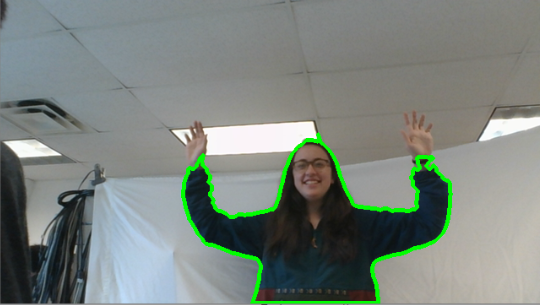Body detection starts with noise reduction. To reduce small imperfections in the image which may affects good detection, we run our initial frame through a Gaussian filter. Since the thresholding operation requires a grayscale image, we also convert from standard RGB to grayscale in this step.

For thresholding, we use Otsu's Binarization. This method of thresholding attempts to separate pixels in the image into two categories, based on brightness. We found that this method works the best when performed on a person wearing dark clothes, standing against a white background. The output of the thresholding operation is a binary image, with darker pixels set to value 1, and lighter pixels set to value 0.

We then draw contours in the image, which finds all non-contiguous blobs of dark colors, and choose the largest blob to be our person. An example of this contour is shown on the right.

#### Elbow Detection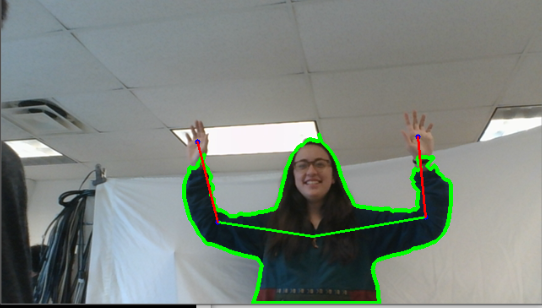To perform elbow detection, we first draw the person contour onto a blank canvas, resulting in the left image below. To restrict the search, we then perform an erosion on the image, which cuts away from the edges. The result is the right image below.

Using a designated chest point, we perform a sweeping angle search, following rays from the chest point to the edge of the body. The longest rays found are the arms, which are highlighted as green lines in the image to the right. Since following the ray returns the intersection point between the ray and edge of the body, we can step backwards from this point into the body, which gives a decent elbow point.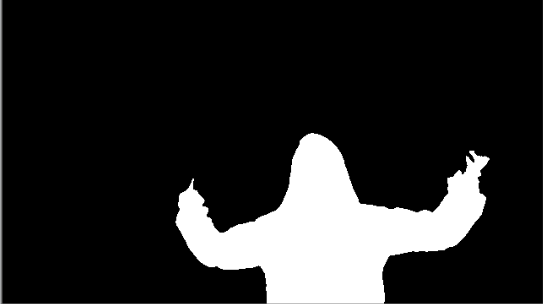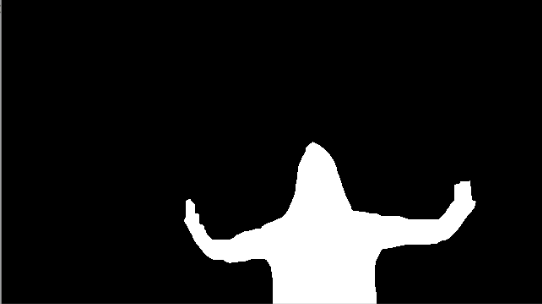Redrawing the contour onto a blank canvas. The right image is after erosion

#### Hand Detection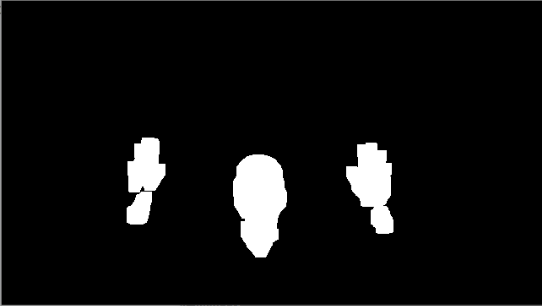According to this paper, a simplified YUV colorspace conversion, coupled with a few simple checks are enough to determine human skin colors. The U component, which is normally a float operation, can be simplified to U = R - G. For human skin, 10 < U < 74. Another simple check, described in this paper, is R > B.

After performing these operations, we are left with a somewhat noisy, but binary image of where human skin is found. To reduce noise, we use the morphological transformation, opening, which is erosion followed by dilation. This removes small patches of noise. We then perform an additional dilation, which closes small holes between larger blobs, and makes contours more accurate. The final image is shown to the left.

Similar to body detection, we draw contours to find all the blobs, and choose the biggest three. From left to right, we label these left hand, head, and right hand. The centroid points of these blobs, found using the moments of the contours, are used instead of the blobs themselves. A small y-axis offset from the head centroid gives us a suitable chest point.

#### Angles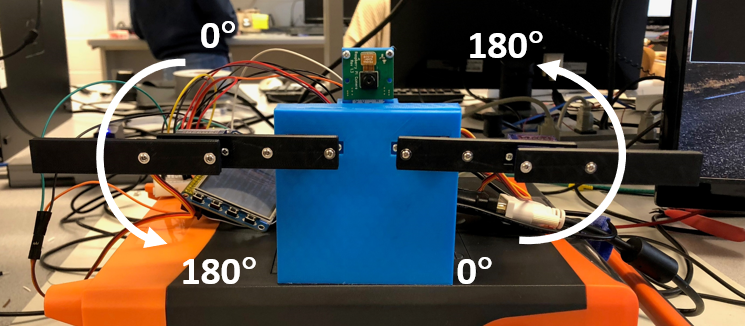There were two angles that we needed to know per arm, the angle of the shoulder joint, and the angle at the elbow. Finding the angle of the shoulder joint was relatively easy since we iterated through angles to find the elbow point. We just returned this angle from the function to use. The angle of the elbow was calculated based on three points: chest, elbow, and hand. From these points we created two vectors and found the angle between them using dot product, cross product and acos. We also needed to find a way to determine which way the angle was opening, up or down. We discovered that the sign of the cross product revealed this information so we returned that value along with the angle.

From here, we needed to map this angle to an angle based on our robot's orientation. So for the left side, straight down was 180 degrees and straight up was 0 degrees. This means that if the arm is straight, the getAngleABC() function would return 180, and this would be mapped to the robots 90 degrees.

### Software Optimization

Without any optimization, the code uses just a single core. On a larger computer this is fine, but on the Raspberry Pi, we need a bit more processing power. The solution is multithreading, as each frame can be processed somewhat individually of other frames. Although Python does offer a Threading module, it is still limited by the Global Interpreter Lock. To get around this, we use the Multiprocessing module, which creates worker processes that bypass the GIL.

Spawning 4 worker threads saturates the Rapsberry Pi's 4 cores, as the main thread mostly waits for asynchronous results. Because the main thread can blocking wait for results from the worker threads, only 4 processes are ever doing meaningful work at one time.

The result is going from about 25-30% CPU usage to about 95% CPU usage at all times. This gives a big increase in throughput, but frame delay still remains the same.

#### Cython

To help with frame delay, speeding up any function that gets run often helps a lot. For our implementation, this function is findWhite, which performs the raytrace described in Elbow Detection. Since it is pure python, fairly simple, and run almost constantly, it is a prime candidate for precompiling using Cython. Just precompiling using Cython gives a ~2x speed increase for the function, but with a couple of small changes, we achieved up to ~100x over pure python. The major changes that we needed to make were just static type declarations and a couple of Cython compiler directives. Below is a comparison between the original code and the code with static declarations:

```def findWhite(fX, fY, l, default, test):
for idx in range(0,len(l)-4,4):
point = l[idx]
if (point<fY and point<fX and
point>0 and point > 0 and
test[int(point),int(point)]) != 255:
return idx
return default
```
```def findWhite(int fX, int fY, int[:,:] l, int default, unsigned char[:,:] test) -> int:
cdef int point0, point1
cdef int idx
for idx in xrange(0,len(l)-4,4):
point0 = l[idx]
point1 = l[idx]
if (point1<fY and point0<fX and
point1>0 and point0 > 0 and
test[point1][point0]) != 255:
return idx
return default
```

Function findWhite. Right is pure python, and left is statically typed.

Adding a few Cython compiler directives helps as well. We disable array bounds checking, array index wraparound, and Nonetype checks.
The result is a python module that can be imported and functions identically to the original, but 100 times faster. Although we don't get 100 times more frames per second, the difference is very noticeable. Coupled with the multiprocessing changes, we're able to run at full resolution without it being unreasonably slow.

## Hardware Design

#### Robot Design

The body of the robot was a relatively simple design. For the front, two rectangular holes were made for the motors to fit through as well as two screw holes for each motor for mounting. We originally 3D printed two triangles to make the body stand up. However we found during testing that these were not very strong and were too light compared to the front of the robot, causing it to easily tip over. We then made the base larger, filling in the area between the triangles. This helped balance he robot as well as created more surface area to epoxy the base on, creating a stronger hold. As for the camera, this was mounted to the top. It protrudes from the back of the body and two screws are used to hold the camera in place. The arms have the same mounting structures for the servos as the main body. The arms also include two other holes to mount the servo horns to connect each arm segment together.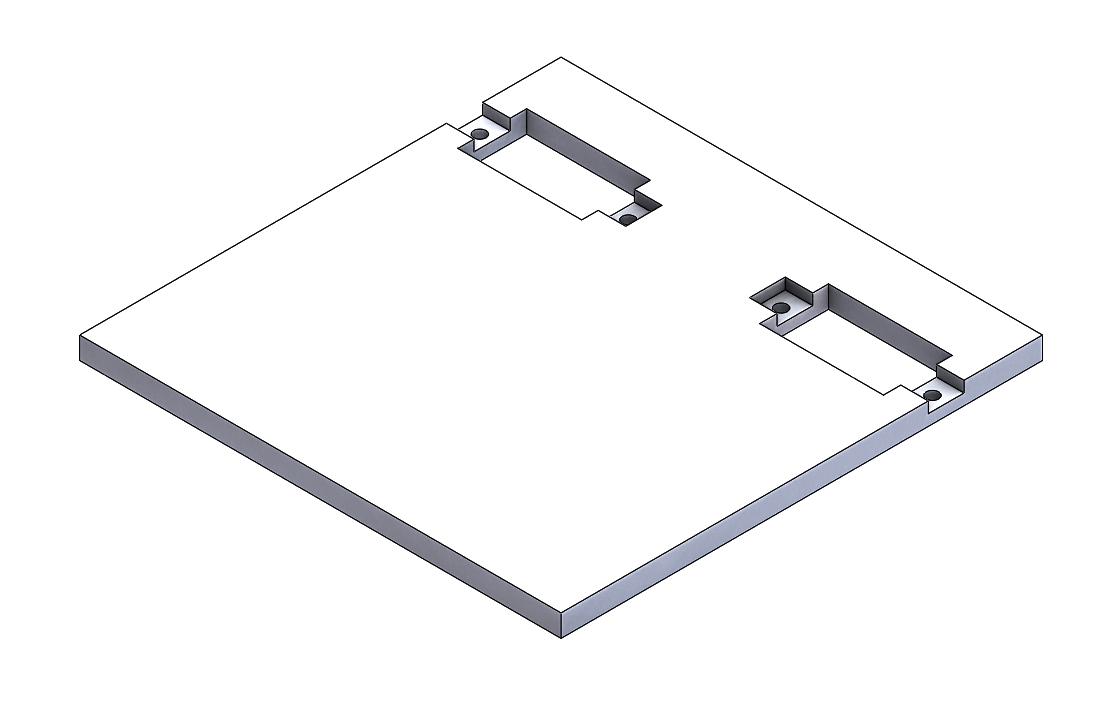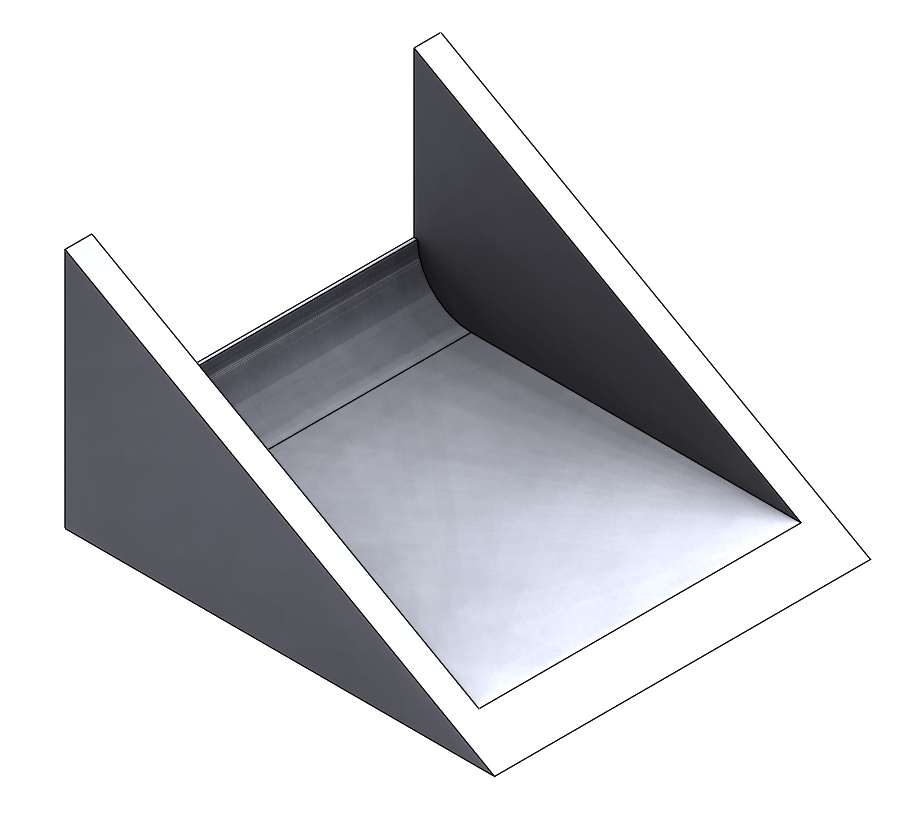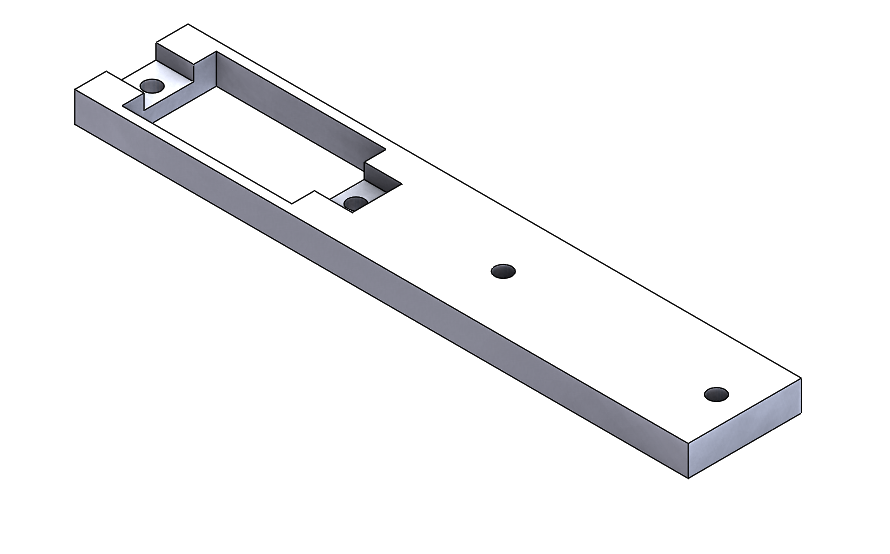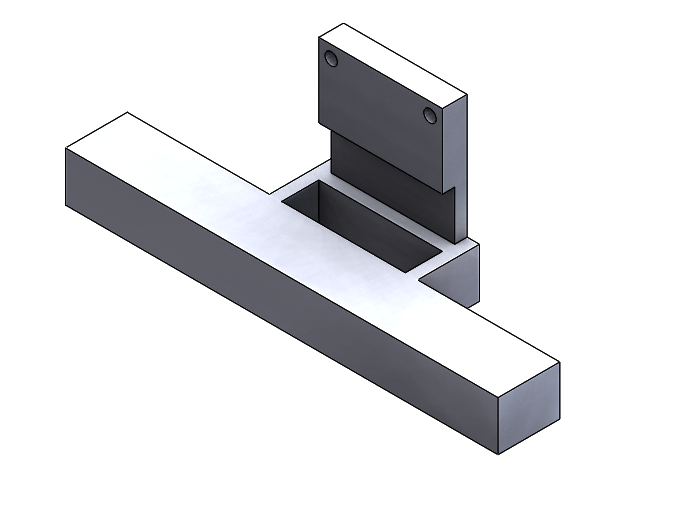#### Servos

We used four micro servos in this project. Each servo was controlled by a different GPIO pin. In order to generate a PWM signal, we used the Pigpio library. This allowed us to use hardware timed PWM signals, creating a stable signal so the motor wouldn't jitter. The motors required 5V for power which we sourced from a power supply. The motor ground connection was wired to the ground of the RasPi so that they would have a common reference ground for the signal. We also added resistors between the RasPi pins and the connection to the servo in order to protect the GPIO pins from current spikes. Once we knew we could control the servos, we found their PWM range for the 180 degrees that we wanted. We found that our lower bound was a pulsewidth of 670, middle was 1550, and the upper bound was 2475. The middle value was used in initialization to attach the arms in the correct orientation, and the upper and lower bounds were used in the mapping function to convert angles to pulsewidths.

## Testing

Software and hardware were mainly tested separately until we had a stable working algorithm since early tracking was quite jumpy. Testing of the servos was relatively simple. We started with testing the control of a software PWM signal. The motors could not hold in once position, which was expected since we knew from earlier labs that a software PWM signal was generally not accurate or stable. We then looked into using hardware PWM signals. The RasPi has two hardware PWM modules that we could have used, however with four motors this was not sufficient. We next turned to the Pigpio library which used hardware timers to create a PWM signal on any of the GPIO pins. Once the timers were set, they would constantly create the signal until updated with a new PWM. With this method, we were able to stably control our servos. We also tested the servos ability to lift weight using thin popsicle sticks found in lab. We wanted to make sure the servos would have enough power to lift an arm with another servo attached. This test was succesful and we proceeded with our servos.

Software was first tested on our own computers where processing would be faster. When we started testing we used a neural network based library, OpenPose. It was extremely accurate, but also ran very slow even on our computers. A single frame took up to 50 seconds on the Raspberry Pi, so we had to move on to different methods. We first experimented with contours and obtaining points from the outline as well as optical flow. While we did use contours to an extent in our final version, we did not use the outline of points that we were originally considering since the group of points didn't tell us a lot of information. As for optical flow, test code was developed to select hands, elbows, and chest points to track. However if a point didn't have a good reference the point was easily lost so we did not continue with this method.

A lot of testing and iteration was performed on the raytracing method for both performance and accuracy, and we put a lot of work into trying to get hand locations using ray tracing. However, we eventually settled on the fact that it wasn't accurate enough, and with the help of an old ECE 5760 project, put together the current hand detection algorithm. However, we were satisfied with its elbow detection, and kept that part for the final code.

Optimization was a slightly bigger challenge, as on our laptops there was no significant drop in framerate from the webcam's 30 frames per second, even for processing-heavy versions of the code. Careful examination of the code, and timing tests on the Cythonized findWhite function helped us achieve better performance on the Raspberry Pi despite no real difference on our laptops.

When choosing where we were going to display the image of the person and their limbs, we wanted to use the PiTFT at first. However, OpenCV is unable to easily output to the PiTFT, and duplicating the desktop to the PiTFT was both unwieldy and took precious processing power. We settled on displaying it on the big screen.

Once confident in the code, we put the system together to test. Software and hardware integrated easily as we already had the angles we needed within the code, all we needed to do was map the values. We first initialized the motors to horizontal so we could attach the arms in the correct orientation. When running the full program, we found that the arms would shake a lot and often wouldn't stay in one place. This was most likely because the motors at the elbows would shake a bit when they moved, and the torque that the shoulders needed to move the upper arm was higher than the motor could provide. In order to rectify this, we reprinted each arm 0.75" shorter than they were previously. After installing the new arms, the robot was much more stable.

We found during testing that the arms would be pretty jumpy, responding to every little change of the detected arms. This wasn't great because the detection was jumpy on it's own. We decided to add a rolling average of angles from the last five frames to help smooth out the movements. We also added bounds to the angles that we could move to so that the system would not overexert itself attempting to ove to an angle over 180 degrees, which would be impossible for it go to.

## Results and Future Work

In the end we were successfully able to create a robot that would accurately mimic a user's movements in real time, as shown in the video above. The only limitation on the system is that the servos are restricted to 180 degrees so we were not able to have sharper angles at the elbow. Overall, we were able to meet the goals that we had set in the project outline.

This project could be further expanded to body movements such as moving up and down or possibly angling the upper body side to side by adding other servos to control the movement in the chest area. By tracking the chest point, up and down motion would be relatively simple. To track side to side motion, another point of reference might be needed. Controlling more servos wouldn't be a problem since any GPIO can use a hardware timer for PWM. The body of the robot would need to be modified to be able to move at all since it is currently just a stand.

## Conclusion

Our project was able to successfully mimic the arm movements of a person. We origninally found a program do detect all body parts and overlay a stick figure onto each frame, however this ran in a neural net which took 40 seconds per frame to process. We were able to achieve a similar program using OpenCV than ran much more effiently so we were able to track movements in real time. On limitation of our system was that the robots arms were limited in their motion since they can only rotate 180 degrees. So while the software can detect sharper angles, our robot could not move to that location.

## Work Distribution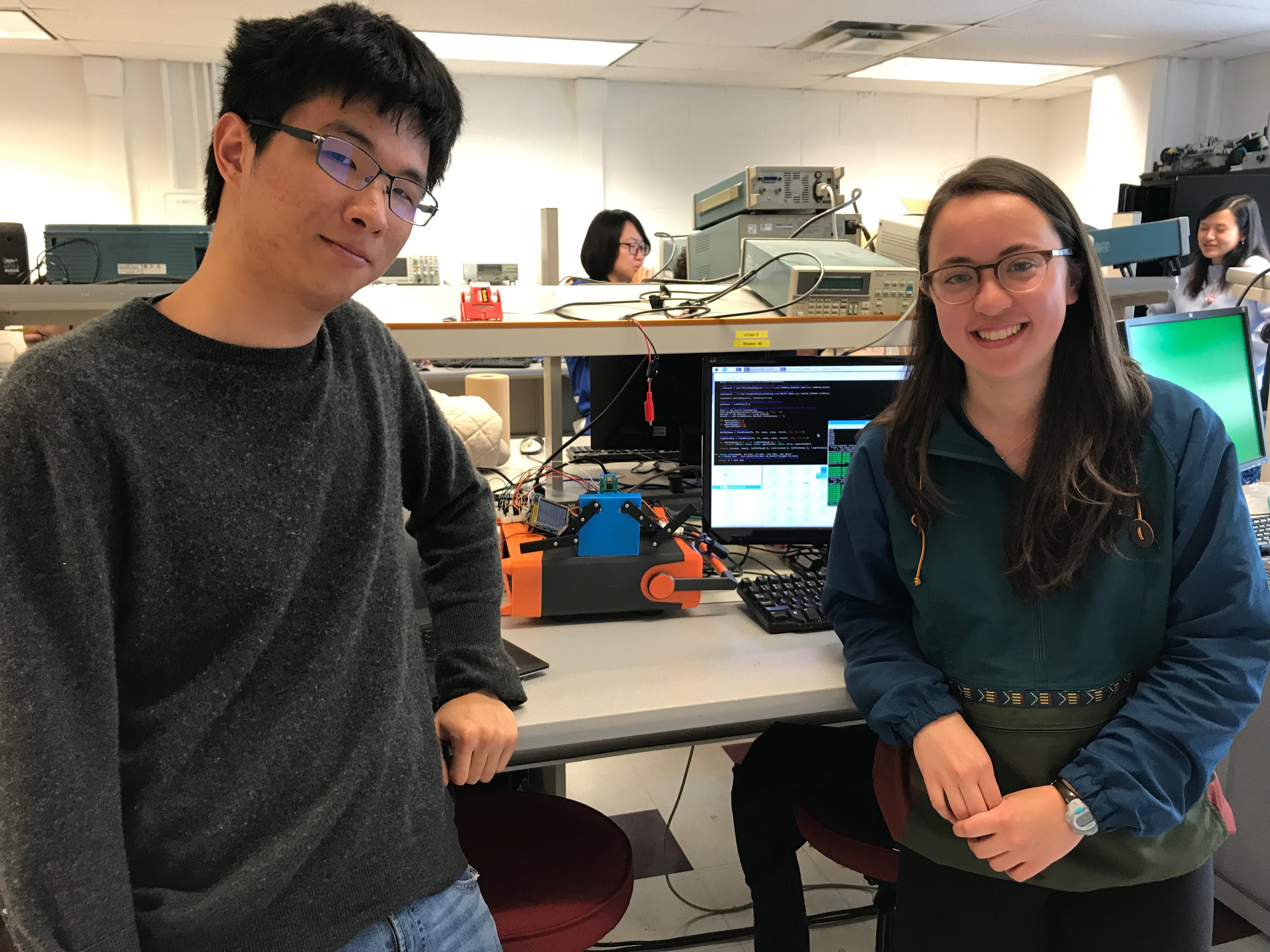#### Project group picture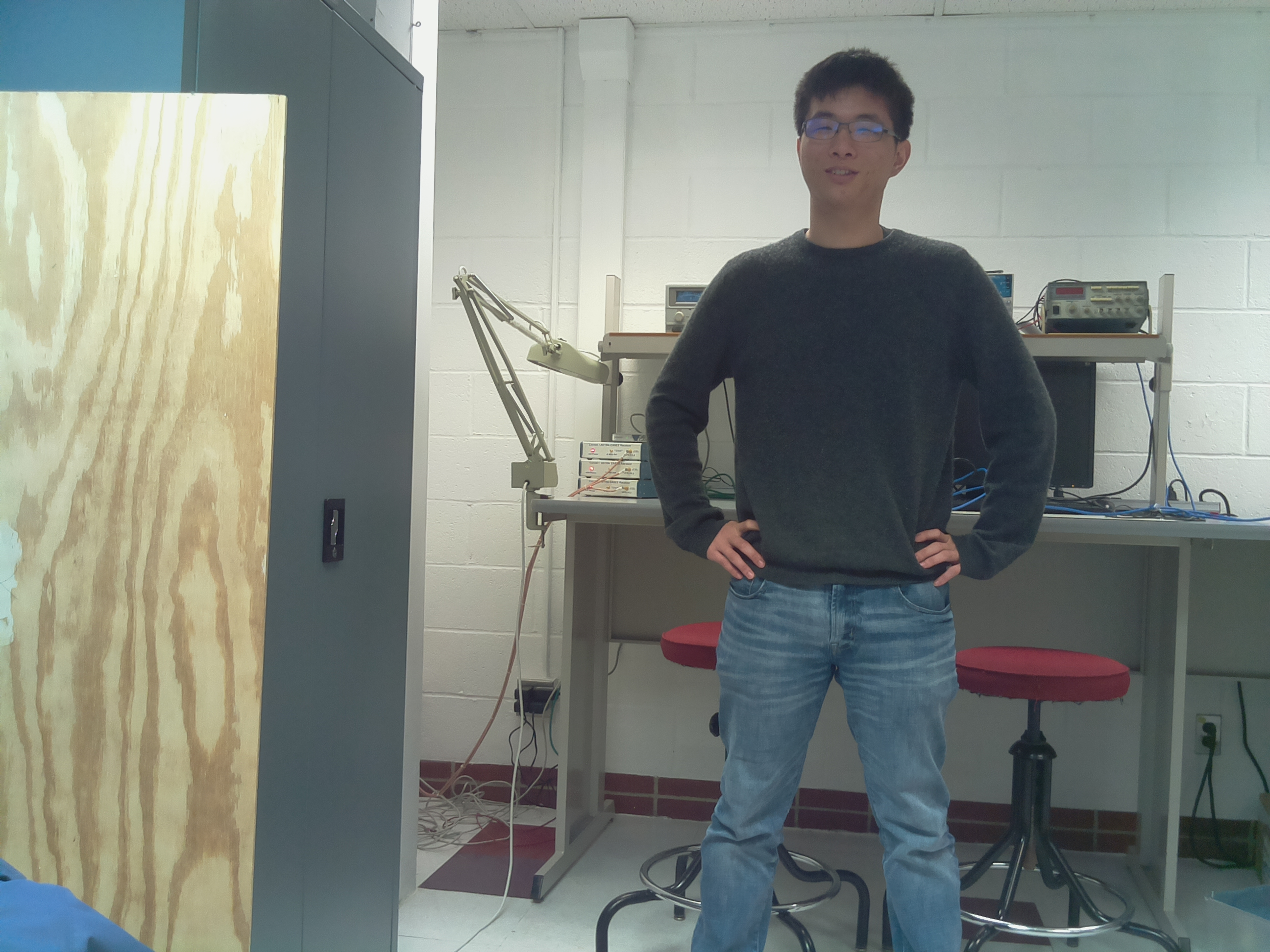### Mark

mkl53@cornell.edu

Software design, system testing### George

Photographer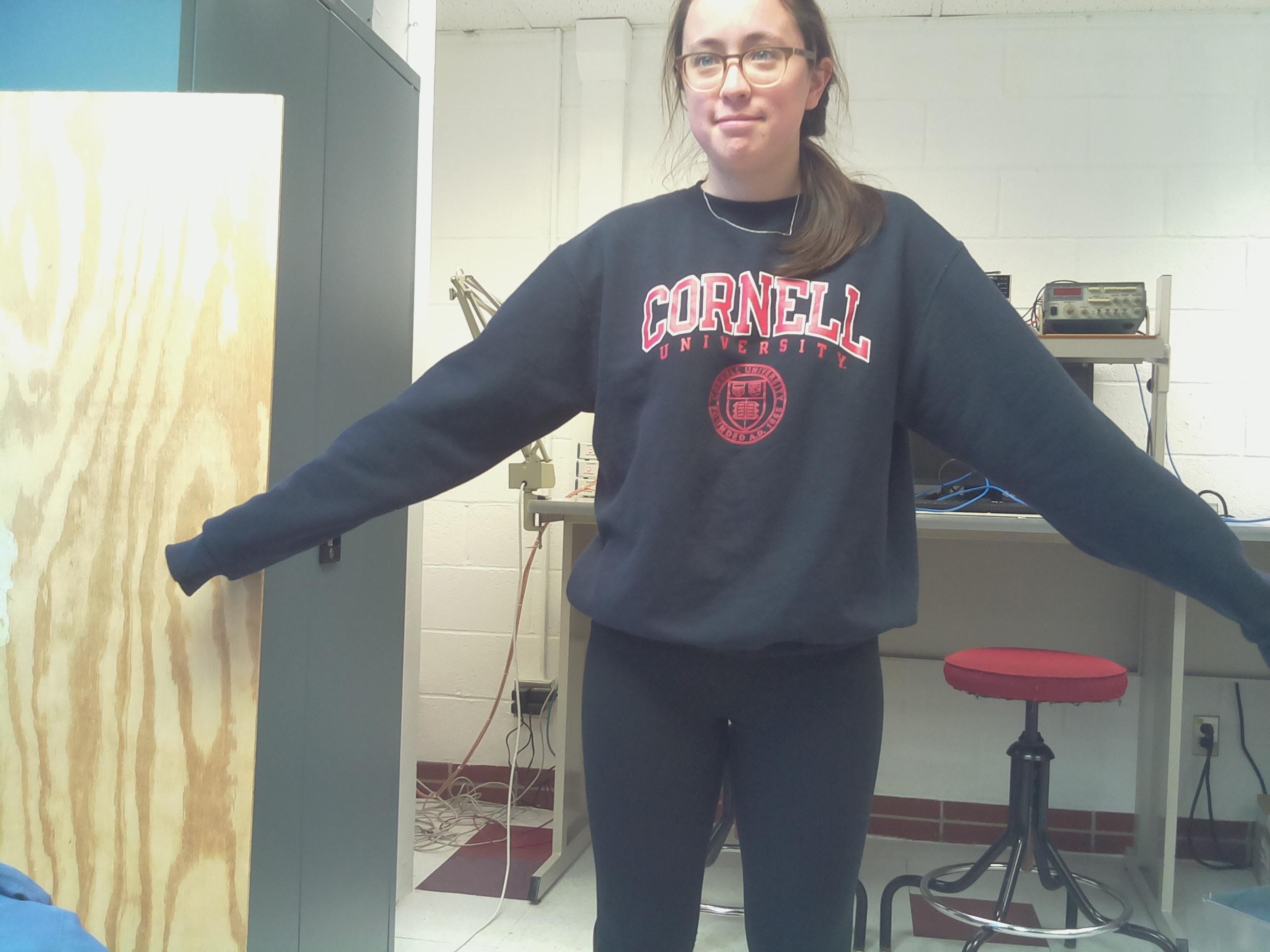### Serena

sk2282@cornell.edu

Robot and hardware design, system testing

## Parts List

Part Quantity Price Total
Pi Cam 1 29.88 29.88
Tower Pro Micro Servos 4 5.95 23.80
Final Total: \$53.78

Note: 3D printed parts were printed by the school and incurred no cost. Wires, resistors, and Raspberry Pi were provided in lab.

## Code Appendix

### setup.py:

```from distutils.core import setup
from distutils.extension import Extension
from Cython.Build import cythonize
setup(ext_modules=cythonize("findWhite.pyx", build_dir="build", annotate=True, compiler_directives={'boundscheck': False, 'wraparound' : False, 'initializedcheck' : False, }),
script_args=['build'],
options={'build':{'build_lib':'.'}})
```

### findWhite.pyx

```def findWhite(int fX, int fY, int[:,:] l, int default, unsigned char[:,:] test) -> int:
cdef int point0, point1
cdef int idx
for idx in xrange(0,len(l)-4,4):
point0 = l[idx]
point1 = l[idx]
if point1<fY and point0<fX and point1>0 and point0 > 0 and test[point1][point0] != 255:
return idx
return default
```

### final.py:

```# Mark Li (mkl53) and Serena Krech (sk2282)
# ECE5725 Spring 2019 - Monday Lab
# Final Project - Mimic Robot
# Note: The following commands must be run before running this program:
#      sudo modprobe2835-bcmv4l2
#      sudo pigpiod
#      python setup.py

import cv2
import numpy as np
import math
import multiprocessing as mp
import os
import pigpio
from findWhite import findWhite

def createLineIterator(P1, P2, img):
imageH = img.shape
imageW = img.shape
P1X = P1
P1Y = P1
P2X = P2
P2Y = P2

#difference and absolute difference between points
#used to calculate slope and relative location between points
dX = P2X - P1X
dY = P2Y - P1Y
dXa = np.abs(dX)
dYa = np.abs(dY)

#predefine numpy array for output based on distance between points
itbuffer = np.empty(shape=(np.maximum(dYa,dXa),3),dtype=np.int)
itbuffer.fill(np.nan)

#Obtain coordinates along the line using a form of Bresenham's algorithm
negY = P1Y > P2Y
negX = P1X > P2X
if P1X == P2X:      #vertical line segment
itbuffer[:,0] = P1X
if negY:
itbuffer[:,1] = np.arange(P1Y - 1,P1Y - dYa - 1,-1)
else:
itbuffer[:,1] = np.arange(P1Y+1,P1Y+dYa+1)
elif P1Y == P2Y:    #horizontal line segment
itbuffer[:,1] = P1Y
if negX:
itbuffer[:,0] = np.arange(P1X-1,P1X-dXa-1,-1)
else:
itbuffer[:,0] = np.arange(P1X+1,P1X+dXa+1)
else:               #diagonal line segment
steepSlope = dYa > dXa
if steepSlope:
slope = dX.astype(np.float32)/dY.astype(np.float32)
if negY:
itbuffer[:,1] = np.arange(P1Y-1,P1Y-dYa-1,-1)
else:
itbuffer[:,1] = np.arange(P1Y+1,P1Y+dYa+1)
itbuffer[:,0] = (slope*(itbuffer[:,1]-P1Y)).astype(np.int) + P1X
else:
slope = dY.astype(np.float32)/dX.astype(np.float32)
if negX:
itbuffer[:,0] = np.arange(P1X-1,P1X-dXa-1,-1)
else:
itbuffer[:,0] = np.arange(P1X+1,P1X+dXa+1)
itbuffer[:,1] = (slope*(itbuffer[:,0]-P1X)).astype(np.int) + P1Y

return itbuffer

def findPeak(fX, fY, sumx, sumy, test, guess, ang1, ang2):
longestU = 0
longestD = 0
angU = [0,0]
ang2U = [0,0]
ang3U = 90
angD = [0,0]
ang2D = [0,0]
ang3D = 90
breakerU=True
breakerD=True
for angle in range(0,max(ang2-guess,guess-ang1)):
if guess + angle <= ang2 and breakerU:
a = angler(fX, fY, guess+angle, sumx, sumy, test);
if a>longestU:
angU = a
ang2U = a
ang3U = guess+angle
longestU = a
elif a < longestU-20:
breakerU=False
if guess - angle >= ang1 and breakerD:
a = angler(fX, fY, guess-angle, sumx, sumy, test);
if a>longestD:
angD = a
ang2D = a
ang3D = guess-angle
longestD = a
elif a < longestD-20:
breakerD=False
if not breakerD and not breakerU:
break
if longestU > longestD:
return (int(angU),int(angU)), (int(ang2U),int(ang2U)), ang3U
else:
return (int(angD),int(angD)), (int(ang2D),int(ang2D)), ang3D

#def findWhite(fX, fY, l, default, test):
#    for idx in range(0,len(l)-4,4):
#        point = l[idx]
#        if point<fY and point<fX and point>0 and point > 0 and test[int(point),int(point)] != 255:
#            return idx
#    return default

def angler(fX, fY, angle, sumx, sumy,test):
if angle == 0:
l=createLineIterator(np.array([sumx, sumy]),np.array([sumx, 0]),test)
elif angle<90:
a = int(tan*sumy)
if a < sumx:
l=createLineIterator(np.array([sumx, sumy]),np.array([sumx-a,0]),test)
else:
l=createLineIterator(np.array([sumx,sumy]),np.array([0,sumy-int(sumx/tan)]),test)
elif angle == 90:
l=createLineIterator(np.array([sumx, sumy]),np.array([0,sumy]),test)
elif angle < 180:
a = int(tan*(sumy-fY))
if a < sumx:
l=createLineIterator(np.array([sumx, sumy]),np.array([sumx-a,fY]),test)
else:
l=createLineIterator(np.array([sumx,sumy]),np.array([0,sumy-int(sumx/tan)]),test)
elif angle == 180:
l=createLineIterator(np.array([sumx, sumy]),np.array([sumx, fY]),test)
elif angle < 270:
a = int(tan*(sumy-fY))
if a < fX-sumx:
l=createLineIterator(np.array([sumx, sumy]),np.array([sumx-a,fY]),test)
else:
l=createLineIterator(np.array([sumx,sumy]),np.array([fX,sumy+int(sumx/tan)]),test)
elif angle == 270:
l=createLineIterator(np.array([sumx, sumy]),np.array([fX, sumy]),test)
else:
a = int(tan*sumy)
if a < fX-sumx:
l=createLineIterator(np.array([sumx, sumy]),np.array([sumx-a,0]),test)
else:
l=createLineIterator(np.array([sumx,sumy]),np.array([fX,sumy+int(sumx/tan)]),test)
idx = findWhite(fX, fY, l, len(l)-1,test)
idx2 = idx-20
if idx2<0:
idx2 = 0
try:
return (math.sqrt((l[idx]-sumx)**2+(l[idx]-sumy)**2), l[idx2], l[idx])
except:
return (0,(sumx, sumy), (sumx, sumy))

# Find arm points - chest, elbows, hands
def armDetect(fX, fY, frame, guessLeft, guessRight):
# Initial blurring for noise reduction
blur = cv2.GaussianBlur(frame,(5,5),0)

# Split the frame into three frames, one for each color
b,g,r = cv2.split(blur)

# Create the U of YUV colorspace. This implementation is simplified compared to other YUV implementations
u = cv2.subtract(r,g)

#for human skin detection, R > B
bminr = cv2.subtract(r,b)

# Human skin 10 < U < 74 AND R > B
mask = cv2.bitwise_and(cv2.inRange(u, 10, 74), cv2.inRange(bminr, 1, 255))

# Perform a small open operation (erode then dilate) to reduce noise
kernel = np.ones((7,7),np.uint8)

# For easy sorting and centroids, draw contours

# Find the 3 largest contours, easiest to just sort it
contours.sort(key=len, reverse=True)

try:
centroids = [(-1,-1),(-1,-1),(-1,-1)] # centroid init

# To get the centroid of contours, use moments
M = cv2.moments(contours)
centroids = (int(M['m10']/M['m00']), int(M['m01']/M['m00']))
M = cv2.moments(contours)
centroids = (int(M['m10']/M['m00']), int(M['m01']/M['m00']))
M = cv2.moments(contours)
centroids = (int(M['m10']/M['m00']), int(M['m01']/M['m00']))
centroids.sort(key=lambda x: x)
except:
return (None, None, None, guessLeft, None, None, guessRight)

# Chest point is somewhere beneath the head for most people so offset y point
sumx = centroids
sumy = centroids + 70

# From now on we need grayscale
gray = cv2.cvtColor(blur,cv2.COLOR_BGR2GRAY)

# otsu thresholding to detect person by contrast to background
_,thresh = cv2.threshold(gray,100,255,cv2.THRESH_BINARY_INV+cv2.THRESH_OTSU)

# Find ALL contours, including internals and whatnot
contours, _ = cv2.findContours(thresh,cv2.RETR_TREE,cv2.CHAIN_APPROX_SIMPLE)

contours.sort(key=len, reverse=True)

# The largest contour is probably the person
contour = contours

# Create two images to raytrace on, one with erosion and one without
test = np.zeros_like(gray)
cv2.drawContours(test,contours, 0, 255, -1)
kernel = np.ones((17,17),np.uint8)
test2 = cv2.erode(test,kernel,iterations = 1)

# Bound guesses for elbow - reduces jumpyness
if guessLeft>130:
guessLeft=130
if guessRight<230:
guessRight=230

# Left arm elbow find
leftElbow = findPeak(fX, fY, sumx, sumy, test2, 90, 35,145)

# Right arm elbow find
rightElbow = findPeak(fX, fY, sumx, sumy, test2, 270, 215,325)

if leftElbow < 20 or rightElbow < 20:
return (None, None, None, guessLeft, None, None, guessRight)

# Return chest, left elbow, left hand, left shoulder angle, right elbow, right hand, right shoulder angle
return ((sumx, sumy), leftElbow, centroids, leftElbow, rightElbow, centroids, rightElbow)

# Mapping function used for angles to PWM
def angle_map(angle, in_min, in_max, out_min, out_max):
x = ((out_max - out_min)/(in_max-in_min))*(angle-in_min)
return x + out_min

# Determine angle between 3 popints
def getAngleABC(a, b, c):
ba = [a - b, a - b]          # Get vectors
bc = [b - c, b - c]

ba_mag = math.sqrt(ba**2 + ba**2)  # Get vector magnitudes
bc_mag = math.sqrt(bc**2 + bc**2)

dot = (ba*bc) + (ba*bc)      # Dot product
cross = ((ba*bc) - (ba*bc))  # Cross product, magnitude

if ba_mag*bc_mag != 0:
cos = dot/(ba_mag*bc_mag)
if abs(cos) > 1:                     # Domain check for acos
return None
else:
return None

# Return correct angle and cross product
return 180- math.degrees(rad), cross    # Sign of cross indicates direction of angle opening

if __name__ == "__main__":

pi = pigpio.pi()                        # Init pi gpio
video_capture = cv2.VideoCapture(0)     # Init video capture

ret,frame = video_capture.read()        # Get first frame
fY,fX,_ = np.shape(frame)

cv2.namedWindow('frame')                # create window

# Initialize variables
avgLeftAngle     = [0, 0, 0, 0, 0]  # holds last 5 left elbow angles
avgRightAngle    = [0, 0, 0, 0, 0]  # holds last 5 right elbow angles
avgLeftShoulder  = [0, 0, 0, 0, 0]  # holds last 5 left shoulder angles
avgRightShoulder = [0, 0, 0, 0, 0]  # holds last 5 right shoulder angles
guessLeft      = 90
guessRight     = 270
leftHand       = (0,0)
rightHand      = (0,0)
avgIdx         = 0                  # index for averaging
lavg           = 0                  # left hand average
ravg           = 0                  # right hand average
lshould_avg    = 0                  # left shoulder average
rshould_avg    = 0                  # right shoulder average

# Multiprocessing
procNum = -1
procs   = [[None, frame.copy()], [None, frame.copy()], [None, frame.copy()], [None, frame.copy()]]
pool    = mp.Pool(processes=4)

# Motor GPIO Pin Definitions
LH_motor = 6
LE_motor = 4
RH_motor = 5
RE_motor = 26

while True:
procNum  = (procNum+1)%4

if procs[procNum] is not None:   # If process is not None
ret = procs[procNum].get()   # Get returned values
if ret is not None:          # If returned actual values
guessLeft = ret
guessRight = ret

cv2.circle(procs[procNum],ret,3,255,-1)               # Left elbow point
cv2.line(procs[procNum], ret, ret, (0,255,0), 2)   # Draw line from chest to left elbow
cv2.circle(procs[procNum],ret,3,255,-1)               # Right elbow point
cv2.line(procs[procNum], ret, ret, (0,255,0), 2)   # Draw line from chest to right elbow

# Store new hand locations if found
if ret is not None:
leftHand = ret
if ret is not None:
rightHand = ret

cv2.circle(procs[procNum], leftHand,3,255,-1)                # Left hand point
cv2.line(procs[procNum], leftHand, ret, (0,0,255), 2)     # Line from left elbow to left hand
cv2.circle(procs[procNum], rightHand,3,255,-1)               # Right hand point
cv2.line(procs[procNum], rightHand, ret, (0,0,255), 2)    # Line from right elbow to right hand

# Find elbow angle and direction angle opens
lelb_ang, l_dir = getAngleABC(ret, ret, leftHand)
relb_ang, r_dir = getAngleABC(ret, ret, rightHand)

# Map returned angles to correct angle 0 - 180 depending on side
if lelb_ang is not None or relb_ang is not None:
if l_dir >=0:   # up
lelb_ang = lelb_ang - 90
else:           # down
lelb_ang = 180 - (lelb_ang-90)

if r_dir >= 0:  #down
relb_ang = 180 - (relb_ang - 90)
else:           # up
relb_ang = relb_ang - 90

# bound the elbow angle due to motor constraints
if lelb_ang < 0:
lelb_ang = 0
elif lelb_ang > 180:
lelb_ang = 180
if relb_ang < 0:
relb_ang = 0
elif relb_ang > 180:
relb_ang = 180

# Add values to rolling average
avgLeftAngle[avgIdx%5] = lelb_ang
avgRightAngle[avgIdx%5] = 180 - relb_ang
avgLeftShoulder[avgIdx%5] = guessLeft
avgRightShoulder[avgIdx%5] = guessRight - 180

# Reinit average values
lavg = ravg = lshould_avg = rshould_avg = 0

# Calculate averages
for i in range(0, 5):
lavg += avgLeftAngle[i]
ravg += avgRightAngle[i]
lshould_avg += avgLeftShoulder[avgIdx%5]
rshould_avg += avgRightShoulder[avgIdx%5]

avgIdx += 1
lavg = lavg/5
ravg = ravg/5
lshould_avg = lshould_avg/5
rshould_avg = rshould_avg/5

# Move the Arms
pi.set_servo_pulsewidth(LE_motor, angle_map(math.floor(lshould_avg), 0, 180, 670, 2475))
pi.set_servo_pulsewidth(LH_motor, angle_map(math.floor(lavg), 0, 180, 670, 2475))
pi.set_servo_pulsewidth(RE_motor, angle_map(math.floor(rshould_avg), 0, 180, 670, 2475))
pi.set_servo_pulsewidth(RH_motor, angle_map(math.floor(ravg), 0, 180, 670, 2475))

cv2.imshow("frame",procs[procNum])   # Display